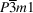International Tables for Crystallography (2016). Vol. A. ch. 1.7, pp. 132-139https://doi.org/10.1107/97809553602060000925

## Contents

• 1.7. Topics on space groups treated in Volumes A1 and E of International Tables for Crystallography  (pp. 132-139)
• 1.7.1. Subgroups and supergroups of space groups  (pp. 132-134) | html | pdf |
• 1.7.1.1. Translationengleiche (or t-) subgroups of space groups  (p. 133) | html | pdf |
• 1.7.1.2. Klassengleiche (or k-) subgroups of space groups  (p. 134) | html | pdf |
• 1.7.1.3. Isomorphic subgroups of space groups  (p. 134) | html | pdf |
• 1.7.1.4. Supergroups  (p. 134) | html | pdf |
• 1.7.2. Relations between Wyckoff positions for group–subgroup-related space groups  (pp. 135-136) | html | pdf |
• 1.7.2.1. Symmetry relations between crystal structures  (p. 135) | html | pdf |
• 1.7.2.2. Substitution derivatives  (p. 135) | html | pdf |
• 1.7.2.3. Phase transitions  (pp. 135-136) | html | pdf |
• 1.7.2.4. Domain structures  (p. 136) | html | pdf |
• 1.7.2.5. Presentation of the relations between the Wyckoff positions among group–subgroup-related space groups  (p. 136) | html | pdf |
• 1.7.3. Relationships between space groups and subperiodic groups  (pp. 136-139) | html | pdf |
• 1.7.3.1. Layer symmetries in three-dimensional crystal structures  (pp. 137-138) | html | pdf |
• 1.7.3.2. The symmetry of domain walls  (pp. 138-139) | html | pdf |
• References | html | pdf |
• Figures
• Fig. 1.7.1.1. Space groupwith t-subgroups of index 2 and 4  (p. 133) | html | pdf |
• Fig. 1.7.2.1. Group–subgroup relation from the aristotype diamond to its hettotype zinc blende  (p. 135) | html | pdf |
• Fig. 1.7.2.2. Group–subgroup relations (Bärnighausen tree) from the ReO3 type to two polymorphic forms of WO3  (p. 136) | html | pdf |
• Fig. 1.7.3.1. The scanning table for the space-group type(164) and orientation orbit (0001), and the structure of cadmium iodide, CdI2  (p. 137) | html | pdf |
• Fig. 1.7.3.2. At the centre is the structure of the cubic phase of barium titanate, BaTiO3, of symmetry type, surrounded by the structures of four of the six single-domain states of the tetragonal phase of symmetry type P4mm  (p. 138) | html | pdf |
• Fig. 1.7.3.3. The domain pair of symmetry P4z/mzmxmxy consisting of the superposition of those two single-domain states of tetragonal symmetry P4zmxmxy shown in Fig. 1.7.3.2  (p. 138) | html | pdf |
• Fig. 1.7.3.4. The domain twin consisting of the two domain states of tetragonal symmetry P4zmxmxy and a domain wall of orientation (010) passing through the origin of the domain twin  (p. 139) | html | pdf |